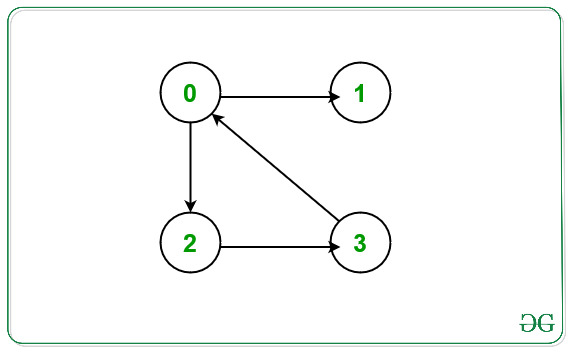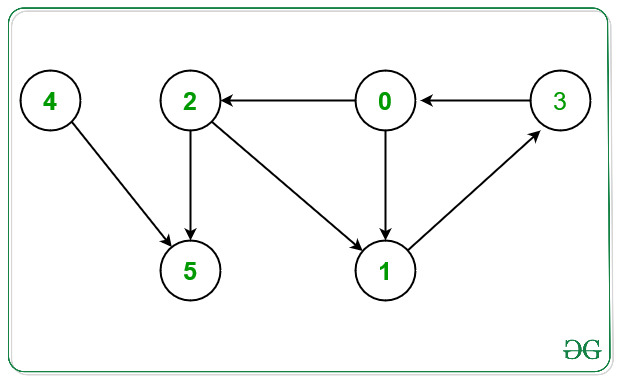# Print Nodes which are not part of any cycle in a Directed Graph

• Difficulty Level : Medium
• Last Updated : 17 Feb, 2021

Given a directed graph G N nodes and E Edges consisting of nodes valued [0, N – 1] and a 2D array Edges[] of type {u, v} that denotes a directed edge between vertices u and v. The task is to find the nodes that are not part of any cycle in the given graph G.

Examples:

Attention reader! Don’t stop learning now. Get hold of all the important DSA concepts with the DSA Self Paced Course at a student-friendly price and become industry ready.  To complete your preparation from learning a language to DS Algo and many more,  please refer Complete Interview Preparation Course.

In case you wish to attend live classes with experts, please refer DSA Live Classes for Working Professionals and Competitive Programming Live for Students.

Input: N = 4, E = 4, Edges[] = { {0, 2}, {0, 1}, {2, 3}, {3, 0} }
Output: 1
Explanation:From the given graph above there exists a cycle between the nodes 0 -> 2 -> 3 -> 0.
Node which doesn’t occurs in any cycle is 1.
Hence, print 1.

Input: N = 6, E = 7, Edges[] = { {0, 1}, {0, 2}, {1, 3}, {2, 1}, {2, 5}, {3, 0}, {4, 5}}
Output: 4 5
Explanation:From the given graph above there exists a cycle between the nodes:
1) 0 -> 1 -> 3 -> 0.
2) 0 -> 2 -> 1 -> 3 -> 0.
Nodes which doesn’t occurs in any cycle are 4 and 5.
Hence, print 4 and 5.

Naive Approach: The simplest approach is to detect a cycle in a directed graph for each node in the given graph and print only those nodes that are not a part of any cycle in the given graph.
Time Complexity: O(V * (V + E)), where V is the number of vertices and E is the number of edges.
Auxiliary Space: O(V)

Efficient Approach: To optimize the above approach, the idea is to store the intermediate node as a visited cycle node whenever any cycle in the given graph. To implement this part use an auxiliary array cyclePart[] that will store the intermediate cycle node while performing the DFS Traversal. Below are the steps:

• Initialize an auxiliary array cyclePart[] of size N, such that if cyclePart[i] = 0, then ith node doesn’t exist in any cycle.
• Initialize an auxiliary array recStack[] of size N, such that it will store the visited node in the recursion stack by marking that node as true.
• Perform the DFS Traversal on the given graph for each unvisited node and do the following:
• Now find a cycle in the given graph, whenever a cycle is found, mark the node in cyclePart[] as true as this node is a part of the cycle.
• If any node is visited in the recursive call and is recStack[node] is also true then that node is a part of the cycle then mark that node as true.
• After performing the DFS Traversal, traverse the array cyclePart[] and print all those nodes that are marked as false as these nodes are not the part of any cycle.

Below is the implementation of the above approach:

## C++

 `// C++ program for the above approach` `#include ``using` `namespace` `std;` `class` `Graph {` `    ``// No. of vertices``    ``int` `V;` `    ``// Stores the Adjacency List``    ``list<``int``>* adj;``    ``bool` `printNodesNotInCycleUtil(``        ``int` `v, ``bool` `visited[], ``bool``* rs,``        ``bool``* cyclePart);` `public``:``    ``// Constructor``    ``Graph(``int` `V);` `    ``// Member Functions``    ``void` `addEdge(``int` `v, ``int` `w);``    ``void` `printNodesNotInCycle();``};` `// Function to initialize the graph``Graph::Graph(``int` `V)``{``    ``this``->V = V;``    ``adj = ``new` `list<``int``>[V];``}` `// Function that adds directed edges``// between node v with node w``void` `Graph::addEdge(``int` `v, ``int` `w)``{``    ``adj[v].push_back(w);``}` `// Function to perform DFS Traversal``// and return true if current node v``// formes cycle``bool` `Graph::printNodesNotInCycleUtil(``    ``int` `v, ``bool` `visited[],``    ``bool``* recStack, ``bool``* cyclePart)``{` `    ``// If node v is unvisited``    ``if` `(visited[v] == ``false``) {` `        ``// Mark the current node as``        ``// visited and part of``        ``// recursion stack``        ``visited[v] = ``true``;``        ``recStack[v] = ``true``;` `        ``// Traverse the Adjacency``        ``// List of current node v``        ``for` `(``auto``& child : adj[v]) {` `            ``// If child node is unvisited``            ``if` `(!visited[child]``                ``&& printNodesNotInCycleUtil(``                       ``child, visited,``                       ``recStack, cyclePart)) {` `                ``// If child node is a part``                ``// of cycle node``                ``cyclePart[child] = 1;``                ``return` `true``;``            ``}` `            ``// If child node is visited``            ``else` `if` `(recStack[child]) {``                ``cyclePart[child] = 1;``                ``return` `true``;``            ``}``        ``}``    ``}` `    ``// Remove vertex from recursion stack``    ``recStack[v] = ``false``;``    ``return` `false``;``}` `// Function that print the nodes for``// the given directed graph that are``// not present in any cycle``void` `Graph::printNodesNotInCycle()``{` `    ``// Stores the visited node``    ``bool``* visited = ``new` `bool``[V];` `    ``// Stores nodes in recursion stack``    ``bool``* recStack = ``new` `bool``[V];` `    ``// Stores the nodes that are``    ``// part of any cycle``    ``bool``* cyclePart = ``new` `bool``[V];` `    ``for` `(``int` `i = 0; i < V; i++) {``        ``visited[i] = ``false``;``        ``recStack[i] = ``false``;``        ``cyclePart[i] = ``false``;``    ``}` `    ``// Traverse each node``    ``for` `(``int` `i = 0; i < V; i++) {` `        ``// If current node is unvisited``        ``if` `(!visited[i]) {` `            ``// Perform DFS Traversal``            ``if` `(printNodesNotInCycleUtil(``                    ``i, visited, recStack,``                    ``cyclePart)) {` `                ``// Mark as cycle node``                ``// if it return true``                ``cyclePart[i] = 1;``            ``}``        ``}``    ``}` `    ``// Traverse the cyclePart[]``    ``for` `(``int` `i = 0; i < V; i++) {` `        ``// If node i is not a part``        ``// of any cycle``        ``if` `(cyclePart[i] == 0) {``            ``cout << i << ``" "``;``        ``}``    ``}``}` `// Function that print the nodes for``// the given directed graph that are``// not present in any cycle``void` `solve(``int` `N, ``int` `E,``           ``int` `Edges[])``{` `    ``// Initialize the graph g``    ``Graph g(N);` `    ``// Create a directed Graph``    ``for` `(``int` `i = 0; i < E; i++) {``        ``g.addEdge(Edges[i],``                  ``Edges[i]);``    ``}` `    ``// Function Call``    ``g.printNodesNotInCycle();``}` `// Driver Code``int` `main()``{``    ``// Given Number of nodes``    ``int` `N = 6;` `    ``// Given Edges``    ``int` `E = 7;` `    ``int` `Edges[] = { { 0, 1 }, { 0, 2 },``                       ``{ 1, 3 }, { 2, 1 },``                       ``{ 2, 5 }, { 3, 0 },``                                 ``{ 4, 5 } };` `    ``// Function Call``    ``solve(N, E, Edges);` `    ``return` `0;``}`

## Java

 `// Java program for above approach``import` `java.util.*;``import` `java.lang.*;``class` `GFG``{` `  ``static` `ArrayList> adj;``  ``static` `int` `V;` `  ``// Function to perform DFS Traversal``  ``// and return true if current node v``  ``// formes cycle``  ``static` `boolean` `printNodesNotInCycleUtil(``    ``int` `v, ``boolean` `visited[],``    ``boolean``[] recStack, ``boolean``[] cyclePart)``  ``{` `    ``// If node v is unvisited``    ``if` `(visited[v] == ``false``)``    ``{` `      ``// Mark the current node as``      ``// visited and part of``      ``// recursion stack``      ``visited[v] = ``true``;``      ``recStack[v] = ``true``;` `      ``// Traverse the Adjacency``      ``// List of current node v``      ``for` `(Integer child : adj.get(v))``      ``{` `        ``// If child node is unvisited``        ``if` `(!visited[child]``            ``&& printNodesNotInCycleUtil(``              ``child, visited,``              ``recStack, cyclePart))``        ``{` `          ``// If child node is a part``          ``// of cycle node``          ``cyclePart[child] = ``true``;``          ``return` `true``;``        ``}` `        ``// If child node is visited``        ``else` `if` `(recStack[child])``        ``{``          ``cyclePart[child] = ``true``;``          ``return` `true``;``        ``}``      ``}``    ``}` `    ``// Remove vertex from recursion stack``    ``recStack[v] = ``false``;``    ``return` `false``;``  ``}` `  ``static` `void` `printNodesNotInCycle()``  ``{` `    ``// Stores the visited node``    ``boolean``[] visited = ``new` `boolean``[V];` `    ``// Stores nodes in recursion stack``    ``boolean``[] recStack = ``new` `boolean``[V];` `    ``// Stores the nodes that are``    ``// part of any cycle``    ``boolean``[] cyclePart = ``new` `boolean``[V];` `    ``// Traverse each node``    ``for` `(``int` `i = ``0``; i < V; i++)``    ``{` `      ``// If current node is unvisited``      ``if` `(!visited[i])``      ``{` `        ``// Perform DFS Traversal``        ``if` `(printNodesNotInCycleUtil(``          ``i, visited, recStack,``          ``cyclePart)) {` `          ``// Mark as cycle node``          ``// if it return true``          ``cyclePart[i] = ``true``;``        ``}``      ``}``    ``}` `    ``// Traverse the cyclePart[]``    ``for` `(``int` `i = ``0``; i < V; i++)``    ``{` `      ``// If node i is not a part``      ``// of any cycle``      ``if` `(!cyclePart[i])``      ``{``        ``System.out.print(i+``" "``);``      ``}``    ``}``  ``}` `  ``// Function that print the nodes for``  ``// the given directed graph that are``  ``// not present in any cycle``  ``static` `void` `solve(``int` `N, ``int` `E,``                    ``int` `Edges[][])``  ``{` `    ``adj = ``new` `ArrayList<>();` `    ``for``(``int` `i = ``0``; i < N; i++)``      ``adj.add(``new` `ArrayList<>());` `    ``// Create a directed Graph``    ``for` `(``int` `i = ``0``; i < E; i++)``    ``{``      ``adj.get(Edges[i][``0``]).add(Edges[i][``1``]);``    ``}` `    ``// Function Call``    ``printNodesNotInCycle();``  ``}``  ` `  ``// Driver function``  ``public` `static` `void` `main (String[] args)``  ``{``    ` `    ``// Given Number of nodes``    ``V = ``6``;` `    ``// Given Edges``    ``int` `E = ``7``;` `    ``int` `Edges[][] = { { ``0``, ``1` `}, { ``0``, ``2` `},``                     ``{ ``1``, ``3` `}, { ``2``, ``1` `},``                     ``{ ``2``, ``5` `}, { ``3``, ``0` `},``                     ``{ ``4``, ``5` `} };`  `    ``// Function Call``    ``solve(V, E, Edges);` `  ``}``}` `// This code is contributed by offbeat`

## Python3

 `# Python3 program for the above approach` `class` `Graph:``    ` `    ``# Function to initialize the graph``    ``def` `__init__(``self``, V):``        ` `        ``self``.V ``=` `V``        ``self``.adj ``=` `[[] ``for` `i ``in` `range``(``self``.V)]``    ` `    ``# Function that adds directed edges``    ``# between node v with node w``    ``def` `addEdge(``self``, v, w):``    ` `        ``self``.adj[v].append(w);``    ` `    ``# Function to perform DFS Traversal``    ``# and return True if current node v``    ``# formes cycle``    ``def` `printNodesNotInCycleUtil(``self``, v, visited,recStack, cyclePart):``    ` `        ``# If node v is unvisited``        ``if` `(visited[v] ``=``=` `False``):``        ` `            ``# Mark the current node as``            ``# visited and part of``            ``# recursion stack``            ``visited[v] ``=` `True``;``            ``recStack[v] ``=` `True``;``    ` `            ``# Traverse the Adjacency``            ``# List of current node v``            ``for` `child ``in` `self``.adj[v]:``            ` `                ``# If child node is unvisited``                ``if` `(``not` `visited[child] ``and` `self``.printNodesNotInCycleUtil(child, visited,recStack, cyclePart)):``                           ` `                    ``# If child node is a part``                    ``# of cycle node``                    ``cyclePart[child] ``=` `1``;``                    ``return` `True``;``    ` `                ``# If child node is visited``                ``elif` `(recStack[child]):``                    ``cyclePart[child] ``=` `1``;``                    ``return` `True``;``                    ` `        ``# Remove vertex from recursion stack``        ``recStack[v] ``=` `False``;``        ``return` `False``;``    ` `    ``# Function that print the nodes for``    ``# the given directed graph that are``    ``# not present in any cycle``    ``def` `printNodesNotInCycle(``self``):``    ` `        ``# Stores the visited node``        ``visited ``=` `[``False` `for` `i ``in` `range``(``self``.V)];``    ` `        ``# Stores nodes in recursion stack``        ``recStack ``=` `[``False` `for` `i ``in` `range``(``self``.V)];``    ` `        ``# Stores the nodes that are``        ``# part of any cycle``        ``cyclePart ``=` `[``False` `for` `i ``in` `range``(``self``.V)]``    ` `        ``# Traverse each node``        ``for` `i ``in` `range``(``self``.V):``        ` `            ``# If current node is unvisited``            ``if` `(``not` `visited[i]):``            ` `                ``# Perform DFS Traversal``                ``if``(``self``.printNodesNotInCycleUtil(``                        ``i, visited, recStack,``                        ``cyclePart)):``                        ` `                    ``# Mark as cycle node``                    ``# if it return True``                    ``cyclePart[i] ``=` `1``;``    ` `        ``# Traverse the cyclePart[]``        ``for` `i ``in` `range``(``self``.V):``        ` `            ``# If node i is not a part``            ``# of any cycle``            ``if` `(cyclePart[i] ``=``=` `0``) :``                ``print``(i,end``=``' '``)``                    ` `# Function that print the nodes for``# the given directed graph that are``# not present in any cycle``def` `solve( N, E, Edges):` `    ``# Initialize the graph g``    ``g ``=` `Graph(N);` `    ``# Create a directed Graph``    ``for` `i ``in` `range``(E):` `        ``g.addEdge(Edges[i][``0``],``                  ``Edges[i][``1``]);  ` `    ``# Function Call``    ``g.printNodesNotInCycle();   ``    ` `    ``# Driver Code``if` `__name__``=``=``'__main__'``:``    ` `    ``# Given Number of nodes``    ``N ``=` `6``;`` ` `    ``# Given Edges``    ``E ``=` `7``;`` ` `    ``Edges ``=` `[ [ ``0``, ``1` `], [ ``0``, ``2` `],``                       ``[ ``1``, ``3` `], [ ``2``, ``1` `],``                       ``[ ``2``, ``5` `], [ ``3``, ``0` `],``                                 ``[ ``4``, ``5` `] ];`` ` `    ``# Function Call``    ``solve(N, E, Edges);``  ` `# This code is contributed by rutvik_56`
Output:
`4 5`

Time Complexity: O(V + E)
Space Complexity: O(V)

My Personal Notes arrow_drop_up